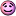CodeGuru Home VC++ / MFC / C++ .NET / C# Visual Basic VB Forums Developer.com

# Thread: Segmentation Fault inside recursive functions

1.Junior MemberJoin Date
Nov 2009
Posts
2

##Segmentation Fault inside recursive functions

Hello Everyone,

I am new to code guru and have been having a major problem with a code assignment I have due in a couple days. I am using several recursive functions to perform operations on trees when I run my program with valgrind I get the following error. One of the functions in which the error occurs is a simple PrintTree function. Any help fixing this function would be greatly appreciated. Thanks! The code that the error occurs in is displayed below the error:

Valgrind command: valgrind run --leak-check=yes --read-var-info=yes --show-unreachable=yes --track-origins=yes

Error:
==2694== Stack overflow in thread 1: can't grow stack to 0xBE39AFFC
==2694==
==2694== Process terminating with default action of signal 11 (SIGSEGV)
==2694== Access not within mapped region at address 0xBE39AFFC
==2694== at 0x804BCBF: std::allocator<Node*>::allocator(std::allocator<Node*> const&) (in /tmp/.users/acd3m4/acd3m4/cs348/assignment5/run)
==2694== Stack overflow in thread 1: can't grow stack to 0xBE39AFF8
==2694==
==2694== Process terminating with default action of signal 11 (SIGSEGV)
==2694== Access not within mapped region at address 0xBE39AFF8
==2694==
==2694== ERROR SUMMARY: 0 errors from 0 contexts (suppressed: 15 from 1)
==2694== malloc/free: in use at exit: 25,800 bytes in 20 blocks.
==2694== malloc/free: 31 allocs, 11 frees, 35,015 bytes allocated.
==2694== For counts of detected errors, rerun with: -v
==2694== searching for pointers to 20 not-freed blocks.
==2694== checked 3,594,140 bytes.
==2694==
==2694== LEAK SUMMARY:
==2694== definitely lost: 0 bytes in 0 blocks.
==2694== possibly lost: 0 bytes in 0 blocks.
==2694== still reachable: 25,800 bytes in 20 blocks.
==2694== suppressed: 0 bytes in 0 blocks.
==2694== Reachable blocks (those to which a pointer was found) are not shown.
==2694== To see them, rerun with: --show-reachable=yes
Segmentation fault

Node Class:

struct Node
{
Node();
~Node();
Node& operator=(Node rhs);
char Operator;
char Type;
double FValue;
double IValue;
vector<Node*> Node_Pointers;
};

Function Call:

PrintTree Function:

void PrintTree(Node CurrentNode, std:fstream& SolutionFile)
{
cout << "Inside Print Tree" << endl;
switch(CurrentNode.Type)
{
case 'f':
if(CurrentNode.FValue < 0)
{
cout << CurrentNode.FValue << endl;
SolutionFile << "(" << CurrentNode.FValue << ")";
SolutionFile << endl;
}
else
{
cout << CurrentNode.FValue << endl;
SolutionFile << CurrentNode.FValue;
SolutionFile << endl;
}
return;
break;

case 'i':
if(CurrentNode.IValue < 0)
{
cout << CurrentNode.IValue << endl;
SolutionFile << "(" << CurrentNode.IValue << ")";
SolutionFile << endl;
}
else
{
cout << CurrentNode.IValue << endl;
SolutionFile << CurrentNode.IValue;
SolutionFile << endl;
}
return;
break;

case 'x':
SolutionFile << "x";
break;

case 'o':
switch(CurrentNode.Operator)
{
case '^':
SolutionFile << "(power(";
PrintTree(*CurrentNode.Node_Pointers, SolutionFile);
SolutionFile << ",";
PrintTree(*CurrentNode.Node_Pointers, SolutionFile);
SolutionFile << "))";
break;

case 'c':
SolutionFile << "(cos(";
PrintTree(*CurrentNode.Node_Pointers, SolutionFile);
SolutionFile << "))";
break;

case 's':
SolutionFile << "(sin(";
PrintTree(*CurrentNode.Node_Pointers, SolutionFile);
SolutionFile << "))";
break;

default:
SolutionFile << "(";
PrintTree(*CurrentNode.Node_Pointers, SolutionFile);
SolutionFile << CurrentNode.Operator ;
PrintTree(*CurrentNode.Node_Pointers, SolutionFile);
SolutionFile << ")";
break;
}
break;
}
return;
}Reply With Quote

2.MemberJoin Date
Nov 2007
Location
Birmingham, England
Posts
157

## Re: Segmentation Fault inside recursive functionsOriginally Posted by crimsonsunI am new to code guru
It appears you're new to programming forums in general. Code tags and correct tabbing go a long way.

Okay the "segmentation fault" is misleading. The error text says "Stack Overflow". This is basically because you can't recurs infinitely. When you call a function (including recursively) all the variables and arguments in the new function call are placed on "the stack" which is a limited size block of memory (typically a couple of MB).

What's happened here is that you've run out of stack memory because you've recursed too many times. This could either be because the tree is too high for you to recurs that many layers, or it is more likely you have a problem with an element's child pointer being set to itself or one of its ancestors. This would be a problem with the tree as it is in memory (and the code that created it).

As a side point, you're passing the current node as an object not by reference or as a pointer. This will take up extra stack space for every call, is slower to run and generally not so commonly done when passing this kind of object to a recursive function.

Hope this helpsReply With Quote

3.Junior MemberJoin Date
Nov 2009
Posts
2

## Re: Segmentation Fault inside recursive functions

Thanks a lot, sorry about the formatting I will fix it next time I didnt notice it didnt paste correctly. I added pass by reference to my functions and it worked just fine, thanks a lot.Reply With Quote

help!, printtree, recursion, segmentation fault, stack overflow####Posting Permissions

• You may not post new threads
• You may not post replies
• You may not post attachments
• You may not edit your posts
•